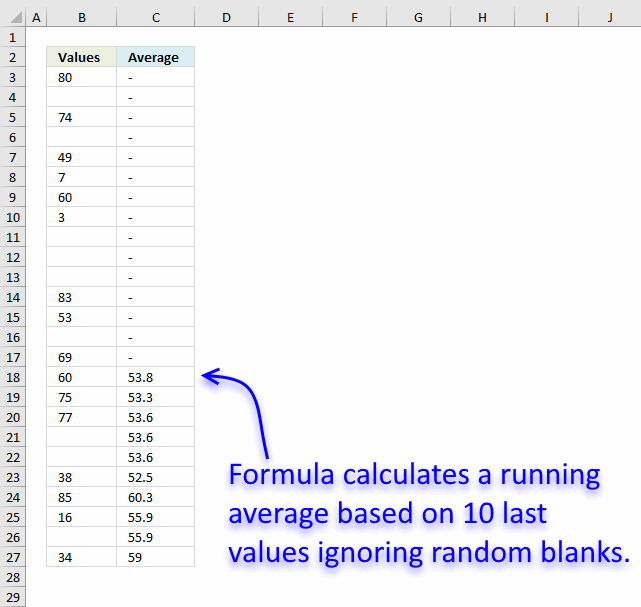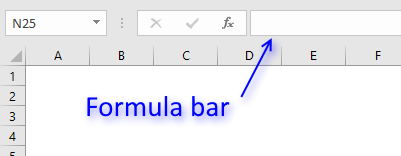Author: Oscar Cronquist Article last updated on January 18, 2019Question:

1. List of data and blank cells in a column which will be added from day to day.
2. There are sometimes blank cells in column.

How to get the average of the 10 most recent data? The average will change from day to day.

This array formula creates a dynamic range, filtering the 10 last data. Adjust cell ranges \$A\$1:\$A\$25 in formula below.

Array formula in cell C3:

=IFERROR(AVERAGE(INDEX(B:B, LARGE(IF(\$B\$3:B3<>"", ROW(\$B\$3:B3), ""), 10)):B3), "-")

#### How to create an array formula

1. Select cell C3
2. Copy (Ctrl + c) array formula
3. Paste (Ctrl + v) array formula to formula bar.4. Press and hold Ctrl + Shift simultaneously.
5. Press Enter once.
6. Release all keys.

### How this array formula works in cell C18

The array formula calculates a cell range to use and then averages the numbers in that cell range.

#### Step 1 - Extract row numbers of not empty cells

The IF function has three arguments, the first one must be a logical expression. If the expression evaluates to TRUE then one thing happens (argument 2) and if FALSE another thing happens (argument 3).

The logical expression contains an expanding cell range that grows when the cell is copied to cells below.

IF(\$B\$3:B18<>"", ROW(\$B\$3:B18), "")

becomes

IF({TRUE; FALSE; TRUE; FALSE; TRUE; TRUE; TRUE; TRUE; FALSE; FALSE; FALSE; TRUE; TRUE; FALSE; TRUE; TRUE}, ROW(\$B\$3:B18), "")

becomes

IF({TRUE; FALSE; TRUE; FALSE; TRUE; TRUE; TRUE; TRUE; FALSE; FALSE; FALSE; TRUE; TRUE; FALSE; TRUE; TRUE}, {3; 4; 5; 6; 7; 8; 9; 10; 11; 12; 13; 14; 15; 16; 17; 18}, "")

and returns {3;"";5;"";7;8;9;10;"";"";"";14;15;"";17;18}.

#### Step 2 - Calculate the 10th largest row number

The LARGE function returns the k-th largest number. LARGE( array, k)

LARGE(IF(\$B\$3:B18<>"", ROW(\$B\$3:B18), ""), 10)

becomes

LARGE({3;"";5;"";7;8;9;10;"";"";"";14;15;"";17;18}, 10)

and returns 3.

#### Step 3 - Use row number to create a cell reference

The INDEX function lets you build a cell reference based on a row and column number.

INDEX(B:B, LARGE(IF(\$B\$3:B3<>"", ROW(\$B\$3:B3), ""), 10))

becomes

INDEX(B:B, 3)

and returns B3.

#### Step 4 - Build complete cell reference

A cell reference may contain two parts separated by a colon.

INDEX(B:B, LARGE(IF(\$B\$3:B3<>"", ROW(\$B\$3:B3), ""), 10)):B18

returns B3:B18.

#### Step 5 - Average values

The AVERAGE function calculates the average based on the cell reference we calculated earlier.

AVERAGE(INDEX(B:B, LARGE(IF(\$B\$3:B3<>"", ROW(\$B\$3:B3), ""), 10)):B18)

becomes

AVERAGE(B3:B18)

and returns 53.8

#### Step 6 - Return - if calculation returns error

The IFERROR fucntion returns "-" if the formula returns an error which it does until 10 numbers are found in the cell range.

### Get the Excel filelast_10_data_average.xlsx